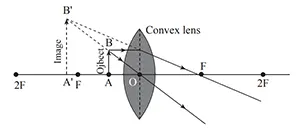# 2 Marks Question

1. How do ciliary muscles focus our eye?
2. Ciliary muscles focus our eye by changing the thickness of the lens inside the eye. It makes the lens thinner to increase the focal length and vice versa.
3. Where should an object be placed in front of a convex lens to get its image magnified, virtual and upright? Draw a ray diagram. Why is a concave lens called a diverging lens?
4. An object should placed between F and O of convex lens to get its image magnified, virtual and upright.
Its ray diagram is shown below:When a parallel beam of light is incident on a concave lens, this beam appears to diverge from a point after the refraction through the lens. So, a concave lens is called a diverging lens.

5. Write any two rules of refraction of light through lens.
6. Rules of refraction of light through lens are:
1. The ray parallel to the principal axis passes through the principal focus after refracting through the lens.
2. The ray passing through the optical center does not deviate.
3. The incident ray passing through the principal focus becomes parallel to the principal axis after refraction through the lens.
7. Why is convex lens called converging lens?
8. The convex lens helps to converge the beam of light to a point after the refraction of light. So, the convex lens is a converging lens.
9. At which point the object should be kept in front of the convex lens to have magnification one. Write two differences between shortsightedness and long-sightedness?
10. When the object is kept in 2F in front of the convex lens then the magnification is one.
Differences between shortsightedness and long-sightedness is shown below in table:
Shortsightedness Longsightedness
The defect of vision in which a person cannot see a distant object but can see a nearby object is called shortsightedness. The defect of vision in which a person can see distant object but cannot see nearby object is called long-sightedness.
Concave lens is used to correct this defect. Convex lens is used to correct this defect.
11. Write two differences between principal focus and focal length.
12. Two differences between principal focus and focal length are:
Principal focus Focal length
It is the point on the principal axis at which the beam of light parallel to the principal axis converge or seems to diverge. It is the distance between the optical center and the principal focus of a lens.
Its symbol is 'F' and has no unit as it is a point. Its symbol is 'f' and unit is meter.
13. Write two differences between converging lens and diverging lens.
14. Two differences between converging lens and diverging lens are shown below in table:
Converging lens Diverging lens
It is thinner at the edge and thicker in the middle. It is thinner at middle and thicker at the edges.
In general, it forms a real image. In general, it forms a virtual image.
Its focus is real. Its focus is virtual.
Its power is taken as positive. Its power is taken as negative.
15. Write two difference between far point and near point?
16. Difference between far point and near point is shown below in table:
Far point Near point
The farthest point from the eye at which an object can be seen clearly by the eye is called the far point of the eye. It is minimum distance for the eye beyond which an eye can accommodate and focus object clearly.
For a normal eye, the far point is at infinity. The near point of a normal eye is 25 cm.